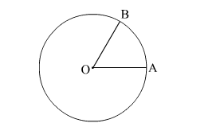# Figure 15.18 shows a sector of a circle of radius r cm containing an angle θ°.Question:

Figure 15.18 shows a sector of a circle of radius r cm containing an angle θ°">θ°θ°. The area of the sector is A cm2 and perimeter of the sector is 50 cm. Prove that

(i) $\theta=\frac{360}{\theta}\left(\frac{25}{r}-1\right)$

(ii) $A=25 r-r^{2}$Solution:

It is given that the radius of circle is $r \mathrm{~cm}$ and angle $\angle A O B=\theta^{\circ}$.(i) We know that the arc length l of a sector of an angle θ in a circle of radius r is

$l=\frac{\theta}{360^{\circ}} \times 2 \pi r$

Perimeter of sector $A O B=O B+O A+\operatorname{arc}$ length $A B$

Now we substitute the value of OB, OA and l to find the perimeter of sector AOB,

Perimeter of sector $A O B=r+r+\frac{\theta}{360^{\circ}} \times 2 \pi r$

$50=2 r\left(1+\frac{\pi \theta}{360^{\circ}}\right)$

$\frac{25}{r}=1+\frac{\pi \theta}{360^{\circ}}$

$\frac{\pi \theta}{360^{\circ}}=\frac{25}{r}-1$

$\theta=\frac{360^{\circ}}{\pi}\left(\frac{25}{r}-1\right)$

(ii) We know that area A of the sector at an angle θ in the circle of radius r is

$A=\frac{\theta}{360^{\circ}} \times \pi r^{2}$. Thus

Area of sector $A O B=\frac{\theta}{360^{\circ}} \pi r^{2}$

Substituting the value of θ,

$A=\frac{\frac{360^{\circ}}{\pi}\left(\frac{25}{r}-1\right)}{360^{\circ}} \pi r^{2}$

$A=\left(\frac{25}{r}-1\right) r^{2}$

$A=25 r-r^{2}$